Get instant live expert help with Excel or Google Sheets“My Excelchat expert helped me in less than 20 minutes, saving me what would have been 5 hours of work!”

#### Post your problem and you’ll get expert help in seconds.

Your message must be at least 40 characters
Our professional experts are available now. Your privacy is guaranteed.

The COUNTIF function in Google Sheets is used to count the number of times a value is found in a selected data range that meets the specified criteria. It is basically a combination of the IF function and COUNT function.

## Using the COUNTIF function in Google Sheets

The COUNTIF function is used where specified criterion is single. It can be divided into two parts for further clarification;

• IF portion of function supplies the criteria to meet for cells or data to count
• COUNT portion of function totals the number of cells that meet that specified criteria

### Basic formula for COUNTIF

The syntax for the COUNTIF function is:

`=COUNTIF(range, criterion)`

Where,

Range is group of cells that we need to search for. Criterion can be any of any of the followings;

• A Number, such as 100
• A text data or text string, such as “Grapes”
• A cell reference where data is located in sheet, such as A1
• An expression, such as “<=100”

There are some rules to follow depending upon data range contains number or text values while specifying the criterion in the COUNTIF function.

If the data range contains numbers:

• Comparison operators, such as > (greater than), >= (greater than equal to), < (less than), <> (not equal to) are used in expression to check the criterion with number. The expression must be enclosed in double quotation marks, such as “<=100”
• Equal sign (=) is not used in an expression to check equal values, such as equal to 100
• In expressions using comparison operators and cell references, the comparison operator is joined to the cell reference using the ampersand (&).
• Comparison operators must be enclosed in double quotation marks, whereas cell references are not enclosed in double quotation marks, such as “>=”&A1

If the data range contains numbers:

• Text string must be enclosed in double quotation marks, such as “Grapes”
• Text strings can contain “?” and “*” wildcard characters. To match one character “?” wildcard is used, and to match multiple contiguous characters “*” wildcard is used, such as “P?T” or “*es”

### How to enter the COUNTIF function in Google Sheets

When you enter any function in Google sheets, an auto-suggest box pops up to follow that function syntax instead of a dialog box in Excel. Like when we will enter COUNTIF function in Google Sheets auto-suggest box will popup; containing syntax, example, summary related to COUNTIF function and explanation of each part of function as shown below.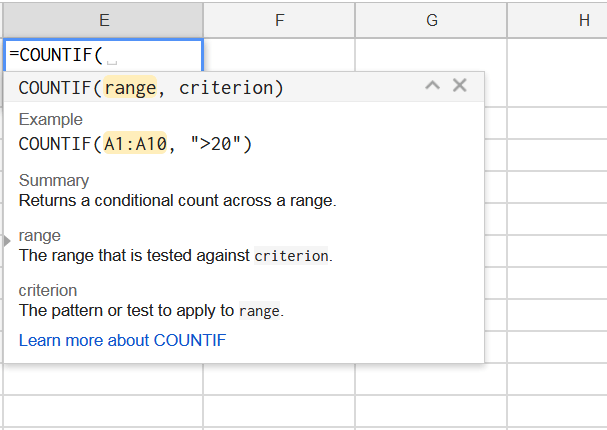1. Keep your cursor in a cell where you want to show your calculations, like cell E1
2. Enter “=” sign and type COUNTIF function in this active cell E1, an auto-suggested box pops up
3. Select the range of cells which you want to text again your criterion
4. Then enter comma “,”
5. Enter criterion expression to apply at selected range
6. Finally, press the ENTER key on the keyboard to enter the closing bracket.

Suppose we have sales dataset of some inventory items and we want to use COUNTIF function in Google Sheets to perform the following calculation on this data set.

### Count of Sales Greater Than \$400

Now we want to test the sales range of B2:B9 again this criterion expression of “>400”. As data range contains numeric values, so we will place the express in double quotation marks, as shown below

You can see the COUNTIF function has tested the selected sales data and counted the values against specified criterion where sales figures are greater than \$400 and returned the result as 2

`=COUNTIF(B2:B9,">400")`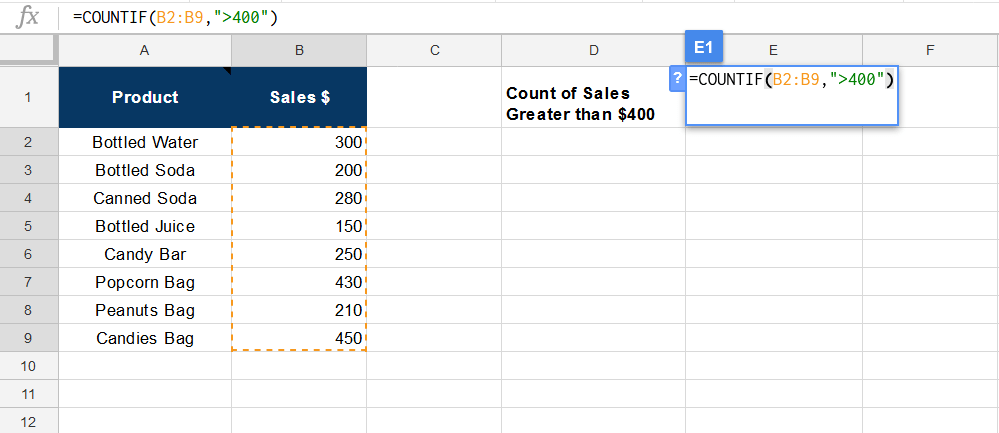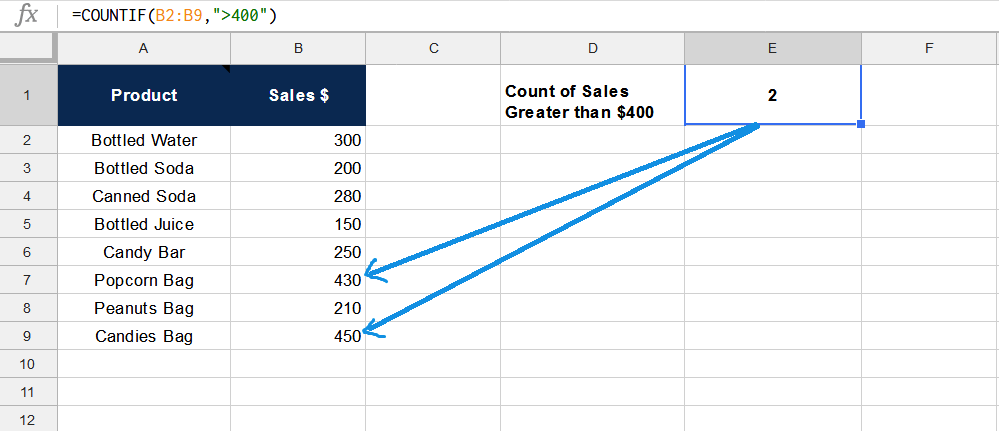Now we will use comparison operator and cell reference in criterion expression to count sales that are greater than the value mentioned in cell reference, such as D2.

We will join comparison operator and cell reference by using an ampersand (&). Comparison operator will be placed in double quotation marks, whereas cell references will not be enclosed in double quotation marks, such as “>”&D2

`=COUNTIF(B2:B9,">"&D2)`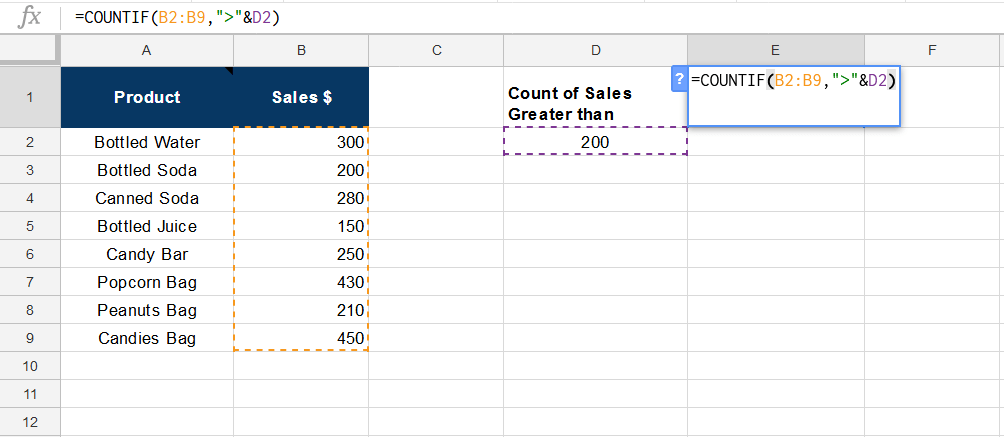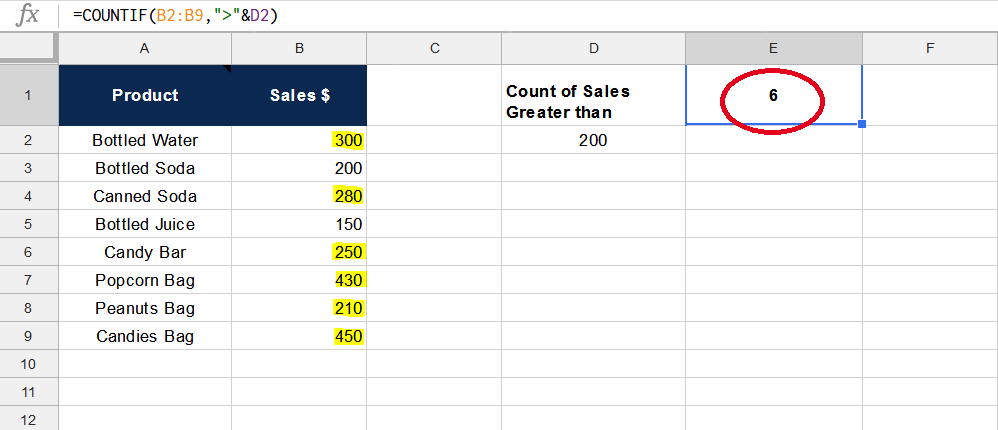### Count of Sales Equal to

IF we want to count the sales figure found in a data range that is exactly equal to a specified value, say \$200, the COUNTIF function will be as follows;

`=COUNTIF(B2:B9,200)`

Here, we will not use the equal sign “=” as comparison operator in criterion expression and criterion numeric value will not be enclosed in double quotation marks.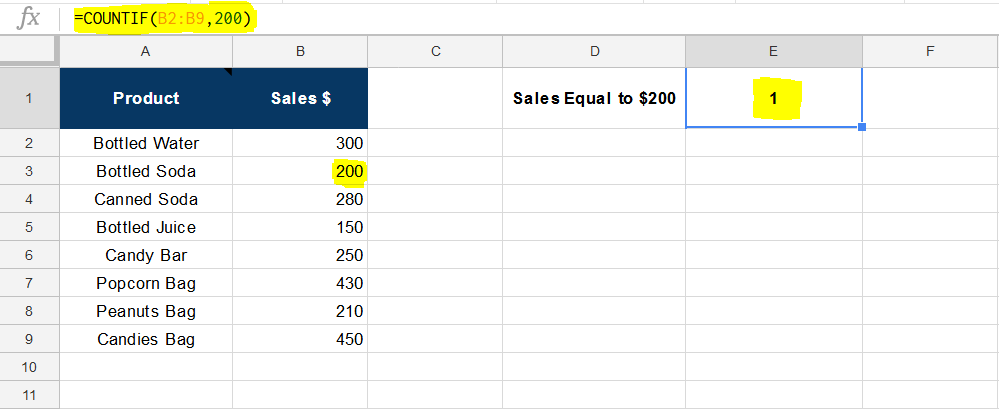### Count of Text value or string

We can count the number of times a text value or text string appears in data range in Google Sheets using COUNTIF functions;

`=COUNTIF(B2:B16,"Utensil")`

Criterion text value will be enclosed in double quotation marks, and cell reference of that value will be used in the formula without double quotation marks, like;

`=COUNTIF(B2:B16,D2)`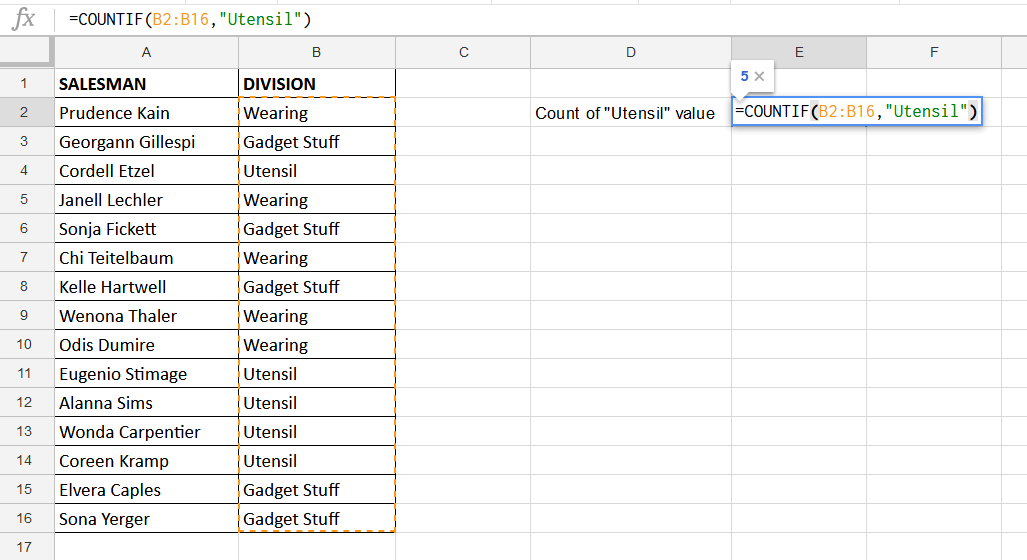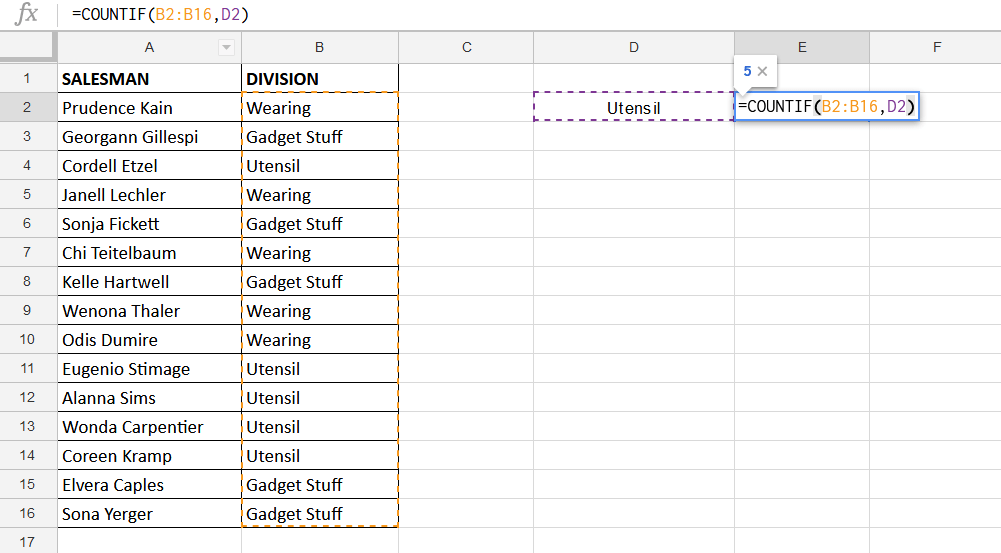Here you can see that COUNTIF has returned the output as number of times (5) a text value is found in data range to test for.

### Count of Text value using Wildcard

You can count any instance of a text value in a data range using wildcards, like “*” and “?”. Wildcard search is not case sensitive and it will count any instance of text value, like

`=COUNTIF(A2:A9,"*Bottled*")`

As criterion text value can be found anywhere in a text string of selected range, then we will place “*” wildcard in start and end of this text value.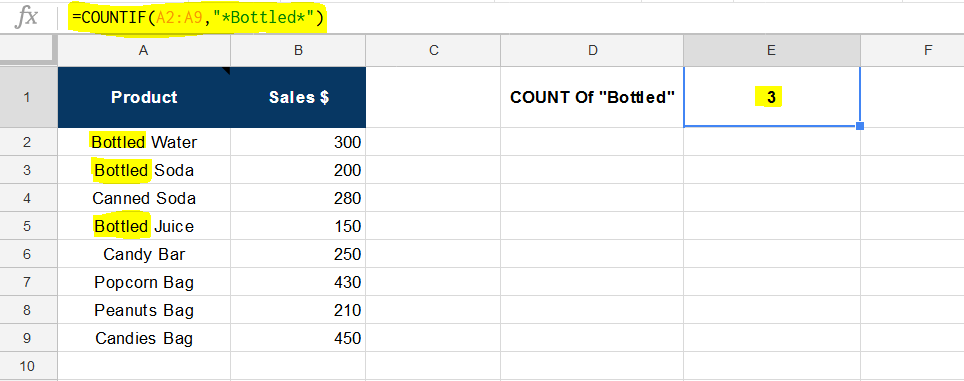IF you have to count the occurrence of text value only at the end of a text string in the data range, then the formula would be;

`=COUNTIF(A2:A9,"*Soda")`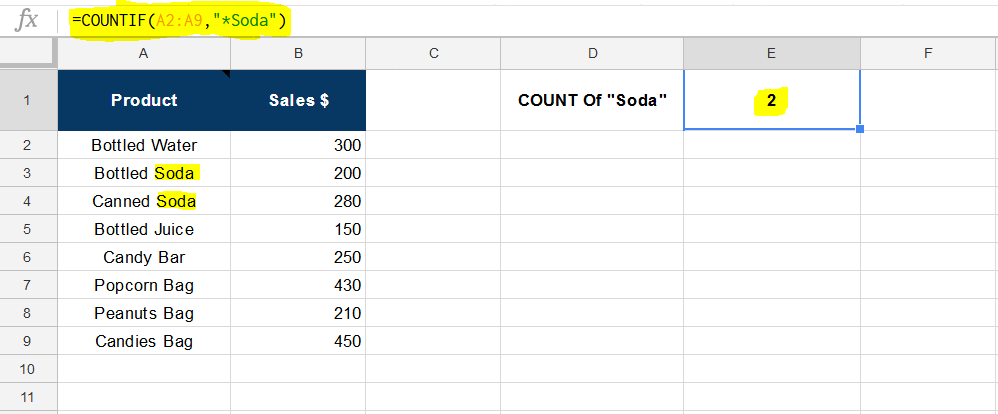Still need some help with Excel formatting or have other questions about Excel? Connect with a live Excel expert here for some 1 on 1 help. Your first session is always free.

### Did this post not answer your question? Get a solution from connecting with the expert.Another blog reader asked this question today on Excelchat:
Related blogs
Solution examplesHello, I am trying to create a total color column? Can anyone help?
Solved by I. A. in 60 minsI have a column of percentages (11 in a column). I want to have a percentage at the bottom of the column that tells me how many of the entries are = to 100%.
Solved by X. E. in 20 minsIs it possible to have a function (like a COUNTIF) that will count sells that meet specific criteria, but the range is limited to the 10 cells in the range that immediately precede a blank cell? So for example, the general range is A1:Z1, but I want the range to be specific to the last ten cells immediately prior to the first blank cell in the general range. Thank you.
Solved by O. F. in 26 minsNeed help putting together formula where it counts the number of sales but only if the average annual premium is above \$360. So a sales = Sum(N:2:N:48)/360....but not greater than COUNTIF(N:2:N:48) ""
Solved by O. J. in 43 minsI used the formula =COUNTIF for cells to define adding the number of cells that have numbers totaling 0 or above. However, When I try using it in another set of columns it is not doing what I need it to. Now, the column that is not working has both colored cells and formula'd numbers within the cells. I dont understand what I am doing wrong, please help!
Solved by C. H. in 20 mins## Subscribe to Excelchat.coAnother blog reader asked this question today on Excelchat: